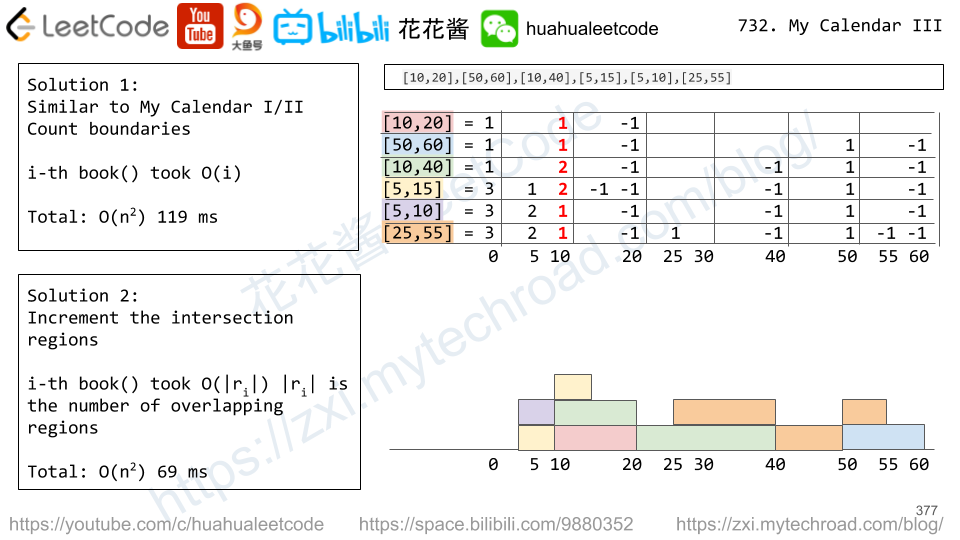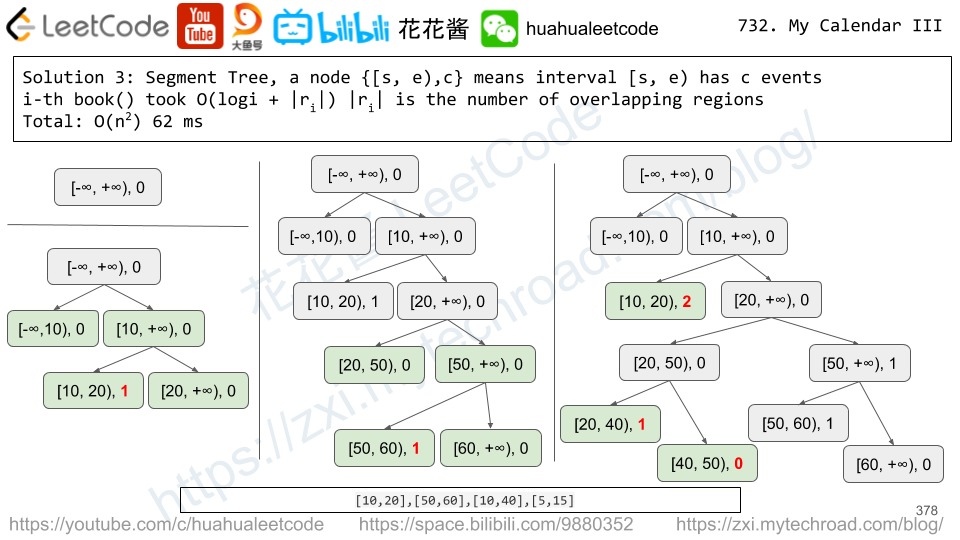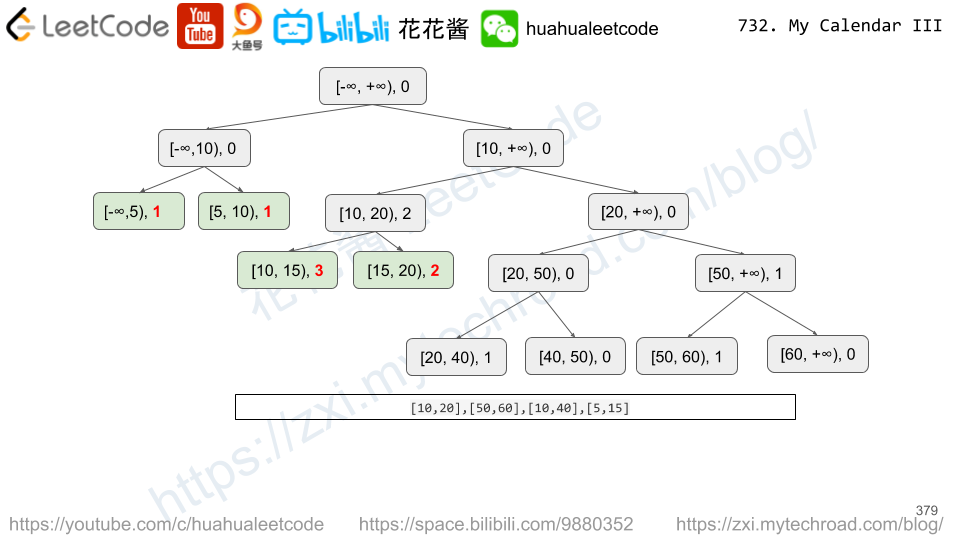# Problem

Given the root node of a binary search tree, return the sum of values of all nodes with value between L and R (inclusive).

The binary search tree is guaranteed to have unique values.

Example 1:

Input: root = [10,5,15,3,7,null,18], L = 7, R = 15
Output: 32


Example 2:

Input: root = [10,5,15,3,7,13,18,1,null,6], L = 6, R = 10
Output: 23


Note:

1. The number of nodes in the tree is at most 10000.
2. The final answer is guaranteed to be less than 2^31.

# Solution: In-order traversal

Time complexity: O(n)

Space complexity: O(n)

# Problem

Given an array A of integers, for each integer A[i] we need to choose either x = -K or x = K, and add x to A[i] (only once).

After this process, we have some array B.

Return the smallest possible difference between the maximum value of B and the minimum value of B.

Example 1:

Input: A = , K = 0
Output: 0
Explanation: B = 


Example 2:

Input: A = [0,10], K = 2
Output: 6
Explanation: B = [2,8]


Example 3:

Input: A = [1,3,6], K = 3
Output: 3
Explanation: B = [4,6,3]


Note:

1. 1 <= A.length <= 10000
2. 0 <= A[i] <= 10000
3. 0 <= K <= 10000

# Solution: Greedy

Sort the array and compare adjacent numbers.

Time complexity: O(nlogn)

Space complexity: O(1)

# Problem

Given an array A of integers, for each integer A[i] we may choose any x with -K <= x <= K, and add xto A[i].

After this process, we have some array B.

Return the smallest possible difference between the maximum value of B and the minimum value of B.

Example 1:

Input: A = , K = 0
Output: 0
Explanation: B = 


Example 2:

Input: A = [0,10], K = 2
Output: 6
Explanation: B = [2,8]


Example 3:

Input: A = [1,3,6], K = 3
Output: 0
Explanation: B = [3,3,3] or B = [4,4,4]


Note:

1. 1 <= A.length <= 10000
2. 0 <= A[i] <= 10000
3. 0 <= K <= 10000

# Solution 0: Brute Force (TLE)

Try all pairs

Time complexity: O(n^2)

Space complexity: O(1)

# Solution 1: Math

Time complexity: O(n)

Space complexity: O(1)

Find the min/max element of the array.

min + k v.s. max – k

ans = max(0, (max – min) – 2 * k))

# Problem

Given an m * n matrix M initialized with all 0‘s and several update operations.

Operations are represented by a 2D array, and each operation is represented by an array with two positiveintegers a and b, which means M[i][j] should be added by one for all 0 <= i < a and 0 <= j < b.

You need to count and return the number of maximum integers in the matrix after performing all the operations.

Example 1:

Input:
m = 3, n = 3
operations = [[2,2],[3,3]]
Output: 4
Explanation:
Initially, M =
[[0, 0, 0],
[0, 0, 0],
[0, 0, 0]]

After performing [2,2], M =
[[1, 1, 0],
[1, 1, 0],
[0, 0, 0]]

After performing [3,3], M =
[[2, 2, 1],
[2, 2, 1],
[1, 1, 1]]

So the maximum integer in M is 2, and there are four of it in M. So return 4.


Note:

1. The range of m and n is [1,40000].
2. The range of a is [1,m], and the range of b is [1,n].
3. The range of operations size won’t exceed 10,000.

# Solution:

Time Complexity: O(n)

Space Complexity: O(1)

C++

Problem:

Implement a MyCalendarThree class to store your events. A new event can always be added.

Your class will have one method, book(int start, int end). Formally, this represents a booking on the half open interval [start, end), the range of real numbers x such that start <= x < end.

K-booking happens when K events have some non-empty intersection (ie., there is some time that is common to all K events.)

For each call to the method MyCalendar.book, return an integer K representing the largest integer such that there exists a K-booking in the calendar.

Your class will be called like this: MyCalendarThree cal = new MyCalendarThree();MyCalendarThree.book(start, end)

Example 1:

MyCalendarThree();
MyCalendarThree.book(10, 20); // returns 1
MyCalendarThree.book(50, 60); // returns 1
MyCalendarThree.book(10, 40); // returns 2
MyCalendarThree.book(5, 15); // returns 3
MyCalendarThree.book(5, 10); // returns 3
MyCalendarThree.book(25, 55); // returns 3
Explanation:
The first two events can be booked and are disjoint, so the maximum K-booking is a 1-booking.
The third event [10, 40) intersects the first event, and the maximum K-booking is a 2-booking.
The remaining events cause the maximum K-booking to be only a 3-booking.
Note that the last event locally causes a 2-booking, but the answer is still 3 because
eg. [10, 20), [10, 40), and [5, 15) are still triple booked.


Note:

• The number of calls to MyCalendarThree.book per test case will be at most 400.
• In calls to MyCalendarThree.book(start, end)start and end are integers in the range [0, 10^9].

Idea:

Similar to LeetCode 731 My Calendar II Use an ordered / tree map to track the number of event at current time.

For a new book event, increase the number of events at start, decrease the number of events at end.

Scan the timeline to find the maximum number of events.# Solution 1: Count Boundaries

Time complexity: O(n^2)

Space complexity: O(n)

# Solution 3: Segment Tree

## Python3

Related Problems:

Mission News Theme by Compete Themes.